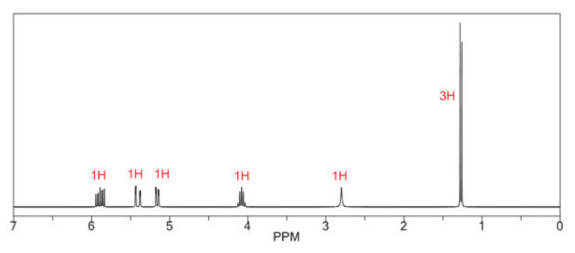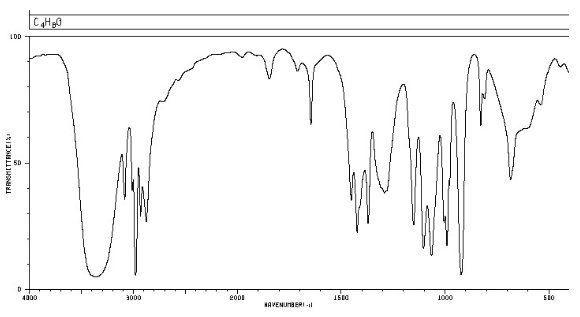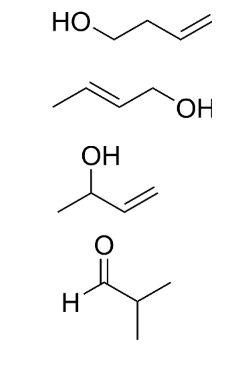# Problem: Select the molecule (C4H8O) that would give the 1H NMR and IR spectra shown below.

###### FREE Expert Solution

Given: C4H8O

IHD:

$\mathbf{IHD}\mathbf{=}\frac{\mathbf{2}\mathbf{\left(}\mathbf{4}\mathbf{\right)}\mathbf{+}\mathbf{2}\mathbf{-}\mathbf{\left[}\mathbf{8}\mathbf{\right]}}{\mathbf{2}}$= 1

IHD = 1 means ring or double bond

99% (447 ratings)###### Problem Details

Select the molecule (C4H8O) that would give the 1H NMR and IR spectra shown below.Frequently Asked Questions

What scientific concept do you need to know in order to solve this problem?

Our tutors have indicated that to solve this problem you will need to apply the Structure Determination without Mass Spect concept. You can view video lessons to learn Structure Determination without Mass Spect. Or if you need more Structure Determination without Mass Spect practice, you can also practice Structure Determination without Mass Spect practice problems.

What professor is this problem relevant for?

Based on our data, we think this problem is relevant for Professor Sacui's class at MEMPHIS.